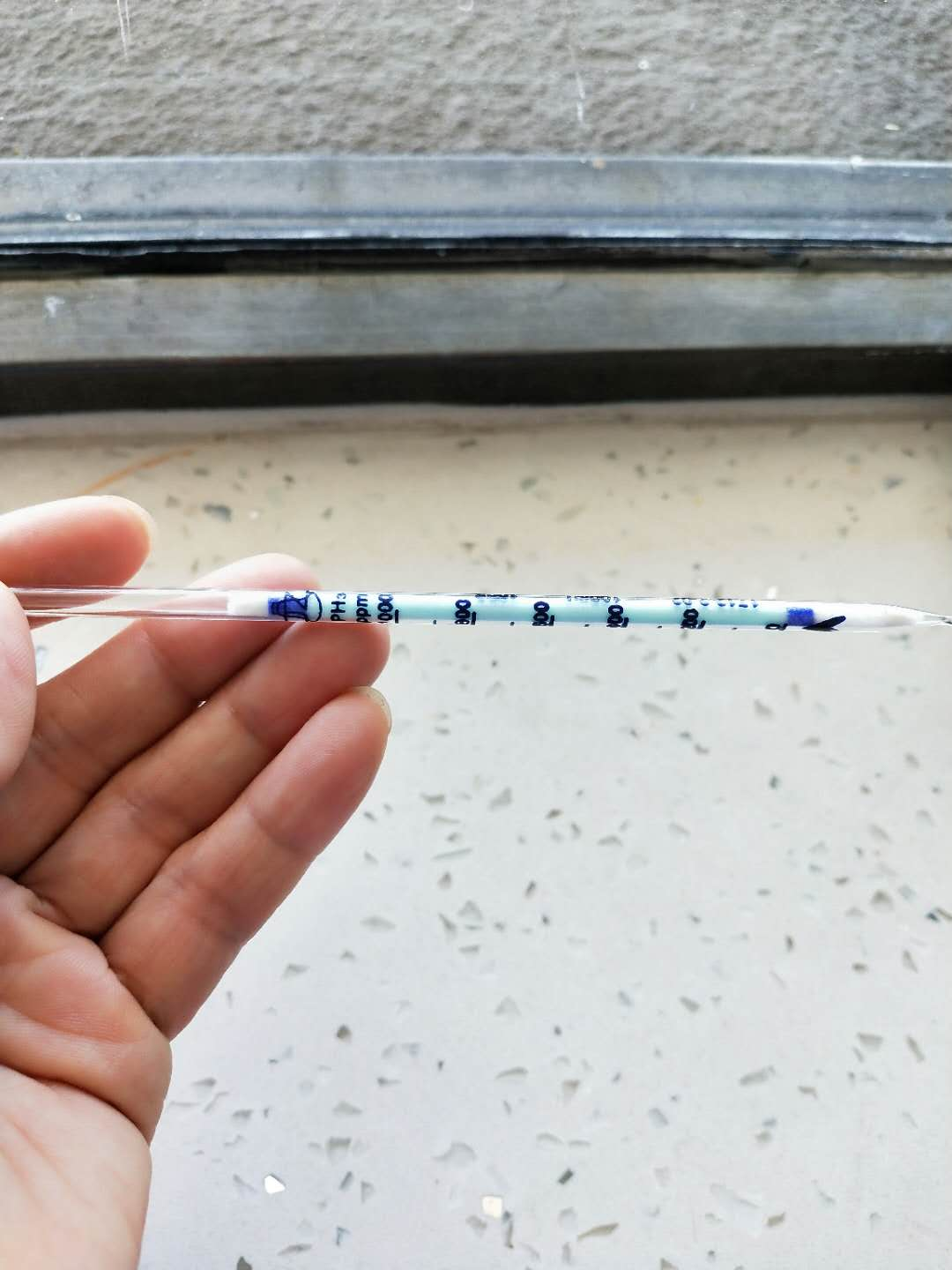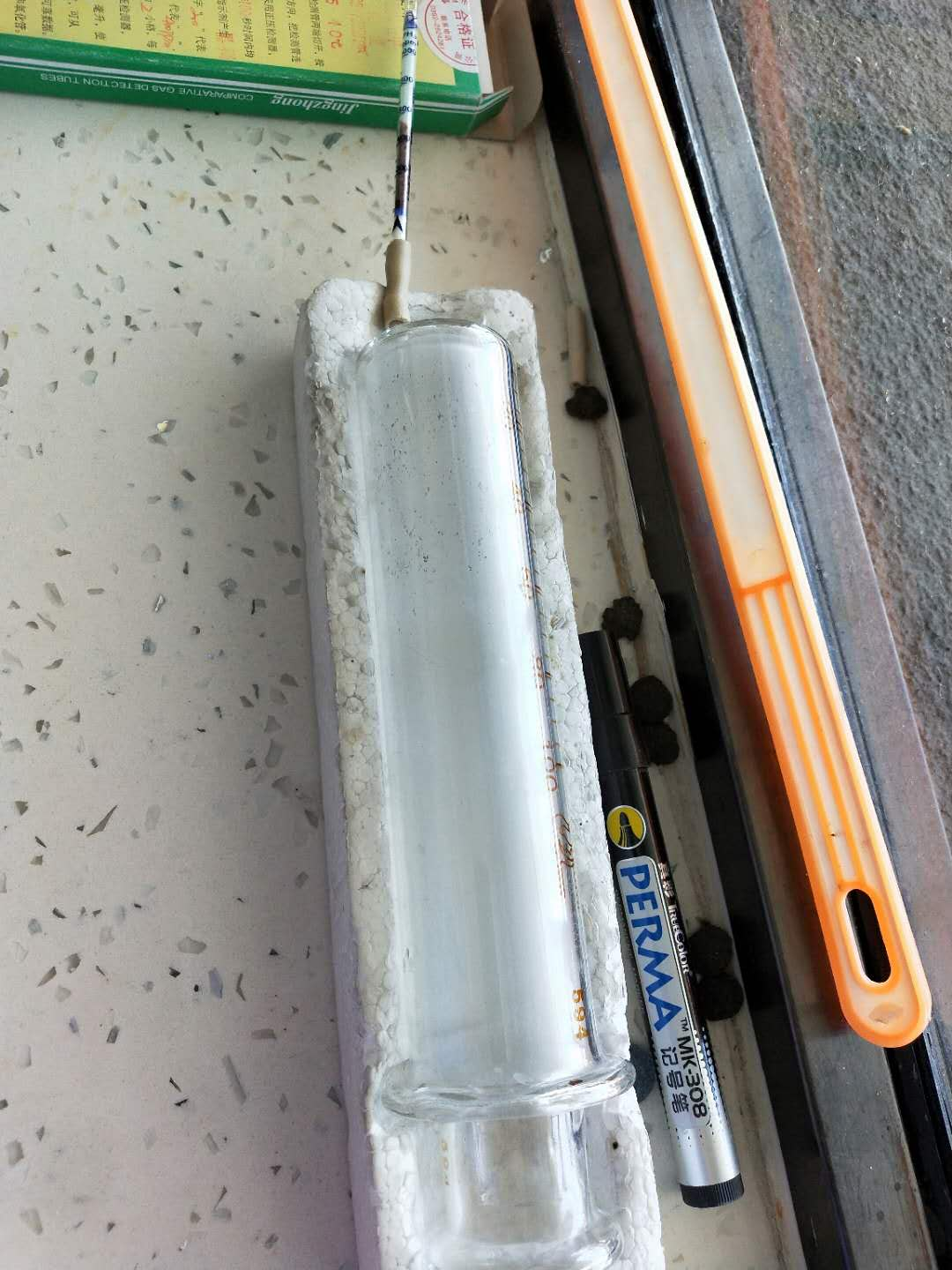The testing of PH3,H2S for Calcium carbide,NGO Chemical Group Ltd Laboratory

# The testing of PH3,H2S for Calcium carbide,NGO Chemical Group Ltd Laboratory

Summary

The testing of PH3,H2S for Calcium carbide,NGO Chemical Group Ltd Laboratory

Complete Reaction of Calcium Carbide and Water Energy in Calcium Carbide: CaC2+2H2O_C2H2_+Ca(OH)2

The volume of acetylene in standard condition can be calculated by draining the gas produced by the reaction and measuring the volume of the discharged water, thus the content of calcium carbide in calcium carbide can be determined.

 序号 1 2 3 4 5 6 导管及仪器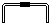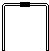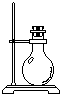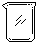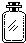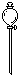每个橡皮塞上都打了两个孔
Complete Reaction of Calcium Carbide and Water Energy in Calcium Carbide: CaC2+2H2O_C2H2_+Ca(OH)2

The volume of acetylene in standard condition can be calculated by draining the gas produced by the reaction and measuring the volume of the discharged water, thus the content of calcium carbide in calcium carbide can be determined.

(1) If the experimental device is assembled with the following instruments and catheters:

Serial number 1 2 3 4 5 6

Catheters and Instruments, Operating Help, Operating Help, Operating Help, Operating Help

Two holes were punched in each rubber plug.

If the gas flow direction is from left to right, the order of direct connection between the above instruments and catheters from left to right (fill in the serial number of each instrument and catheter) is as follows: ___________________

(2) After the instrument is connected, the following operations are performed during the experiment (each operation is performed only once):

1. Weigh a certain amount of calcium carbide and place it in Instrument 3. Tighten the rubber plug.

(2) Check the airtightness of the device.

3. Inject appropriate amount of water into instruments 6 and 5.

(4) When instrument 3 is restored to room temperature, the volume of water in instrument 4 is measured (water in conduit 2 is neglected).

(5) Slowly open the piston of Instrument 6 so that water drops down one by one. When no gas occurs, close the piston.